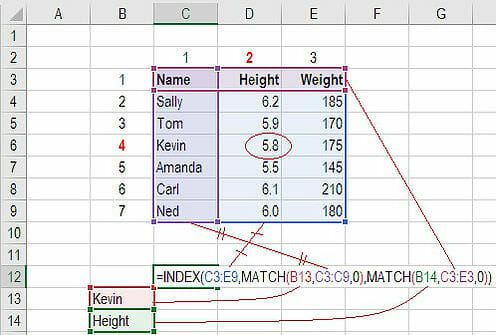# Advanced Excel Formulas – 10 Formulas You Must Know!-YRP.COM.NP

## 10 Advanced Excel Formulas You Must Know

Every financial analyst spends more time in Excel than they may care to admit.  Based on years and years of experience, we have compiled the most important and advanced Excel formulas that every world-class financial analyst must know.

### Excel Ebook

Download the free ebook for over 200 pages of important lessons on Excel shortcuts, formulas, and functions.

### 1. INDEX MATCH

Formula: =INDEX(C3:E9,MATCH(B13,C3:C9,0),MATCH(B14,C3:E3,0))

This is an advanced alternative to the VLOOKUP or HLOOKUP formulas (which have several drawbacks and limitations).  INDEX MATCH is a powerful combination of Excel formulas that will take your financial analysis and financial modeling to the next level.

INDEX returns the value of a cell in a table based on the column and row number.

MATCH returns the position of a cell in a row or column.

Here is an example of the INDEX and MATCH formulas combined together.  In this example, we look up and return a person’s height based on their name.  Since name and height are both variables in the formula, we can change both of them!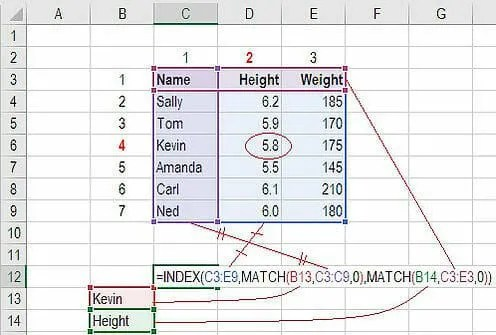For a step-by-step explanation or how to use this formula, please see our free guide on how to use INDEX MATCH in Excel.

### 2. IF combined with AND / OR

Formula: =IF(AND(C2>=C4,C2<=C5),C6,C7)

Anyone who’s spent a great deal of time doing various types of financial models knows that nested IF formulas can be a nightmare.  Combining IF with the AND or the OR function can be a great way to keep formulas easier to audit and easier for other users to understand.  In the example below, you will see how we used the individual functions in combination to create a more advanced formula.

For a detailed breakdown of how to perform this function in Excel please see our free guide on how to use IF with AND / OR.### 3. OFFSET combined with SUM or AVERAGE

Formula: =SUM(B4:OFFSET(B4,0,E2-1))

The OFFSET function on its own is not particularly advanced, but when we combine it with other functions like SUM or AVERAGE we can create a pretty sophisticated formula.  Suppose you want to create a dynamic function that can sum a variable number of cells.  With the regular SUM formula, you are limited to a static calculation, but by adding OFFSET you can have the cell reference move around.

How it works:  To make this formula work, we substitute the ending reference cell of the SUM function with the OFFSET function.  This makes the formula dynamic and the cell referenced as E2 is where you can tell Excel how many consecutive cells you want to add up. Now we’ve got some advanced Excel formulas!

Below is a screenshot of this slightly more sophisticated formula in action.As you see, the SUM formula starts in cell B4, but it ends with a variable, which is the OFFSET formula starting at B4 and continuing by the value in E2 (“3”), minus one.  This moves the end of the sum formula over 2 cells, summing 3 years of data (including the starting point).  As you can see in cell F7, the sum of cells B4:D4 is 15, which is what the offset and sum formula gives us.

### 4. CHOOSE

Formula: =CHOOSE(choice, option1, option2, option3)

The CHOOSE function is great for scenario analysis in financial modeling.  It allows you to pick between a specific number of options, and return the “choice” that you’ve selected.  For example, imagine you have three different assumptions for revenue growth next year: 5%, 12%, and 18%.  Using the CHOOSE formula you can return 12% if you tell Excel you want choice #2.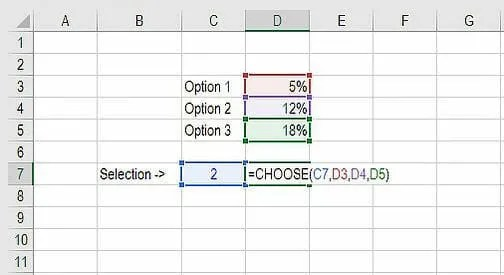To see a video demonstration, check out our Advanced Excel Formulas Course.

### 5. XNPV and XIRR

Formula: =XNPV(discount rate, cash flows, dates)

If you’re an analyst working in investment banking, equity research, financial planning & analysis (FP&A), or any other area of corporate finance that requires discounting cash flows, then these formulas are a lifesaver!

Simply put, XNPV and XIRR allow you to apply specific dates to each individual cash flow that’s being discounted.  The problem with Excel’s basic NPV and IRR formulas is that they assume the time periods between cash flow are equal.  Routinely, as an analyst, you’ll have situations where cash flows are not timed evenly, and this formula is how you fix that.For a more detailed breakdown, see our free IRR vs XIRR formulas guide as well as our XNPV guide.

### 6. SUMIF and COUNTIF

Formula: =COUNTIF(D5:D12,”>=21″)

These two advanced formulas are great uses of conditional functions.  SUMIF adds all cells that meet certain criteria, and COUNTIF counts all cells that meet certain criteria. For example, imagine you want to count all cells that are greater than or equal to 21 (the legal drinking age in the U.S.) to find out how many bottles of champagne you need for a client event.  You can use COUNTIF as an advanced solution, as shown in the screenshot below.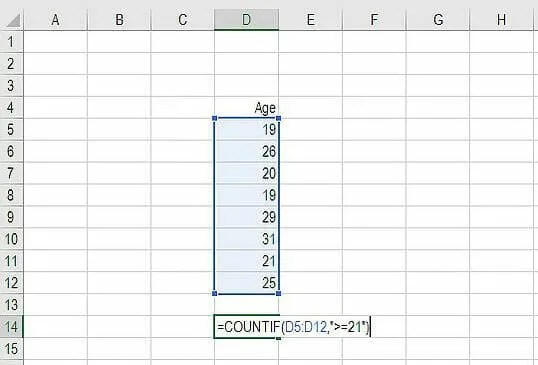In our advanced Excel course, we break these formulas down in even more detail.

### 7. PMT and IPMT

Formula: =PMT(interest rate, # of periods, present value)

If you work in commercial banking, real estate, FP&A or any financial analyst position that deals with debt schedules, you’ll want to understand these two detailed formulas.

The PMT formula gives you the value of equal payments over the life of a loan.  You can use it in conjunction with IPMT (which tells you the interest payments for the same type of loan), then separate principal and interest payments.

Here is an example of how to use the PMT function to get the monthly mortgage payment for a \$1 million mortgage at 5% for 30 years.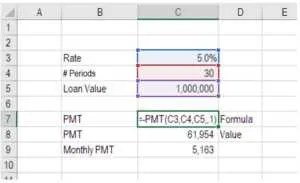### 8. LEN and TRIM

Formulas: =LEN(text) and =TRIM(text)

The above formulas are a little less common, but certainly very sophisticated ones.  They are great for financial analysts who need to organize and manipulate large amounts of data.  Unfortunately, the data we get is not always perfectly organized and sometimes, there can be issues like extra spaces at the beginning or end of cells.

The LEN formula returns a given text string as the number of characters, which is useful when you want to count how many characters there are in some text.

In the example below, you can see how the TRIM formula cleans up the Excel data.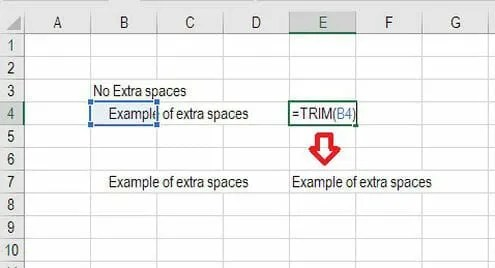### 9. CONCATENATE

Formula: =A1&” more text”

Concatenate is not really a function on its own – it’s just an innovative way of joining information from different cells and making worksheets more dynamic.  This is a very powerful tool for financial analysts performing financial modeling (see our free financial modeling guide to learn more).

In the example below, you can see how the text “New York” plus “, “ is joined with “NY” to create “New York, NY”. This allows you to create dynamic headers and labels in worksheets. Now, instead of updating cell B8 directly, you can update cells B2 and D2 independently.  With a large data set, this is a valuable skill to have at your disposal.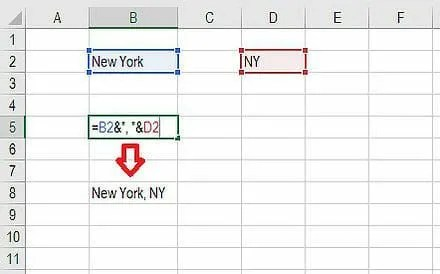### 10. CELL, LEFT, MID and RIGHT functions

These advanced Excel functions can be combined to create some very advanced and complex formulas to use.  The CELL function can return a variety of information about the contents of a cell (such as its name, location, row, column, and more).  The LEFT function can return text from the beginning of a cell (left to right), MID returns text from any start point of the cell (left to right), and RIGHT returns text from the end of the cell (right to left).

Below is an illustration of the three formulas in action.

To see how they can be combined in a powerful way with the CELL function, we break it down for you step by step in our advanced Excel formulas class.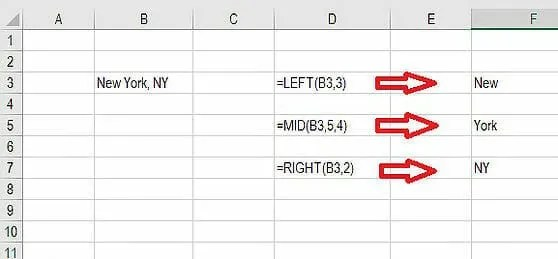### More Excel Formulas Training

We hope these top 10 advanced Excel formulas have been helpful for you. They should go a long way to improving your financial analysis and financial modeling skills.

Below are more CFI resources to help you become an Excel power user:

• Excel Formulas Cheat Sheet
• Excel Keyboard Shortcuts
• Free Excel Crash Course